Latest Banking jobs   »   Quantitative Aptitude Quiz For LIC AAO...

# Quantitative Aptitude Quiz For LIC AAO 2023- 22nd January

Q1. The product of the age of Bhagat and Rahu is 240. If twice the age of Rahu is more than Bhagat’s age by 4 years. Then what is the age of Rahu?
(a) 12 years
(b) 10 years
(c) 15 years
(d) 8 years
(e) 20 years

Q2. A part of Rs. 9600 is invested at a 5% annual return, while the remainder is invested at 3% annual return. If the annual income from both portion is the same, what is the total income from the two investments.
(a) Rs. 380
(b) Rs. 320
(c) Rs. 440
(d) Rs. 360
(e) Rs. 520

Q3. Present average age of a couple is 29 years after 2 year and 4 year respectively a boy child and a girl child is born. Find the average age of family after 8 year of present time?
(a) 18
(b) 19
(c) 20
(d) 21
(e) 22

Q4. The present age of Bhagat and Abhi are in ratio of 9 : 8 respectively. After 10 years the ratio of their ages will be 10 : 9. What is the difference in their present age ?
(a) 8 years
(b) 6 years
(c) 12 years
(d) 4 years
(e) 10 years

Q5. The ratio of the ages of Ram and Rahim 10 years ago was 1 : 3. The ratio of their ages five years hence will be 2 : 3. Then, the ratio of their present ages is :
(a) 1 : 2
(b) 3 : 5
(c) 3 : 4
(d) 2 : 5
(e) None of these

Q6. Veer’s grandfather was 11 times older to him 6 years ago and he will be 3 times of veer’s age 18 years from now. Six years hence form now, what will be the ratio of Veer’s age to that of his grandfather?
(a) 5 : 11
(b) 11 : 5
(c) 4 : 15
(d) 13 : 3
(e) 3 : 13

Q7. Five year hence, average age of A, B and C is 27. Six years ago ratio of age of A and B is 6 : 7. B is 8 years younger than C. then, find the difference between C’s age and A’s age ?
(a) 14
(b) 12
(c) 10
(d) 8
(e) 6

Q8. Average age of Akshita, Sonakshi and Abhishek is 32 after 4 year. Ratio of present age of Askhita and Abhishek is 3 : 4. If sum of present age of Akshita and sonakshi is 52. Then find the difference between age of Sonakshi and Abhishek.
(a) 6
(b) 8
(c) 3
(d) 5
(e) 4

Q9. Difference between CI and SI on a sum for 3 year at 20% p.a. is 176, Find the simple interest on the sum after 2 year at 10% p.a.?
(a) 225
(b) 250
(c) 275
(d) 300
(e) 350

Q10. The average age of Ram and his son, five years ago was 27.5. Ram’s present age is five years more than thrice of his son’s present age. Find ratio of their present ages?
(a) 14 : 9
(b) 15 : 4
(c) 20 : 7
(d) 10 : 3
(e) 9 : 5

Q11. If on a sum the difference b/w simple interest and compound interest at rate of 12% p.a. for two years is Rs. 51.84. Then find the amount a man will get if he invested same sum for 2 years at 10% p.a. compounded annually?
(a) Rs. 4598
(b) Rs. 3993
(c) Rs. 4114
(d) Rs. 4235
(e) Rs. 4356

Q12. Raj invested Rs. 15000 in scheme ‘A’ at rate of 18% p.a. simple interest for 3 years and Riya invested Rs. 18000 in scheme ‘B’ which offers 15% p.a. compound interest in 2 years. Find the difference between the interest earned from these two schemes?
(a) Rs. 2295
(b) Rs. 2400
(c) Rs. 2500
(d) Rs. 2345
(e) Rs. 2100

Q13. Average present age of Ritu and Priya is 22. After 8 years, ratio b/w priya’s age to ritu’s age is 7 : 8, then find the ratio of Ritu’s age to priya’s age two years ago.
(a) 12 : 11
(b) 11 : 9
(c) 8 : 7
(d) 9 : 7
(e) 13 : 11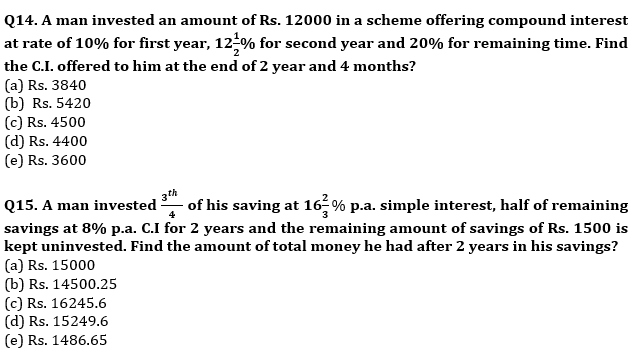Solutions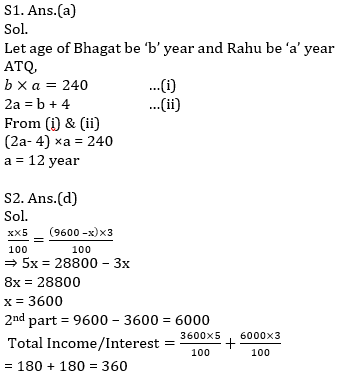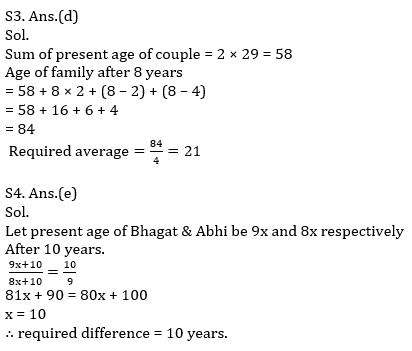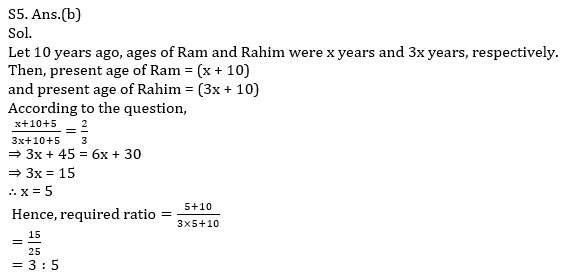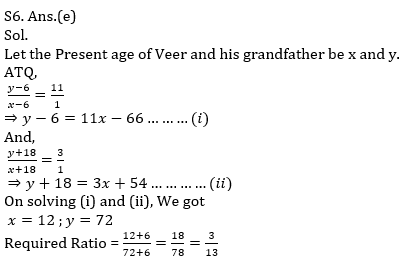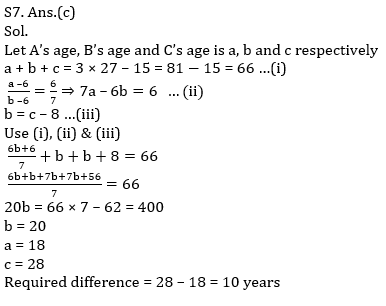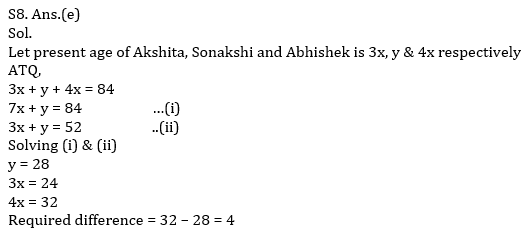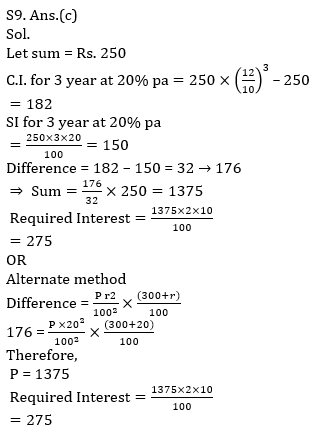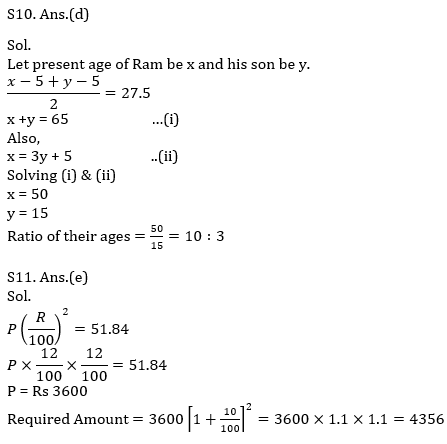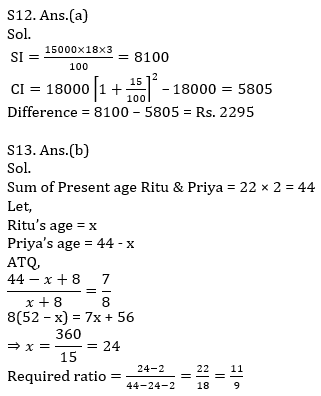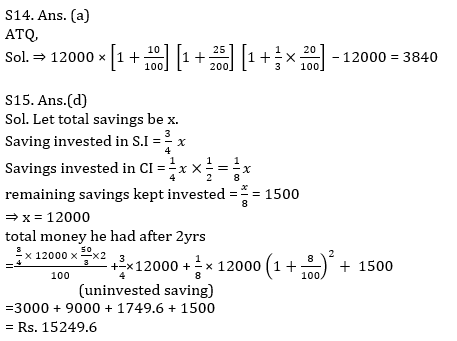## FAQs

### When will the LIC AAO Prelims exam be held?

The LIC AAO Prelims exam will be held on 17th & 20th of February 2023 (tentatively).

#### Congratulations!Union Budget 2023-24: Free PDF